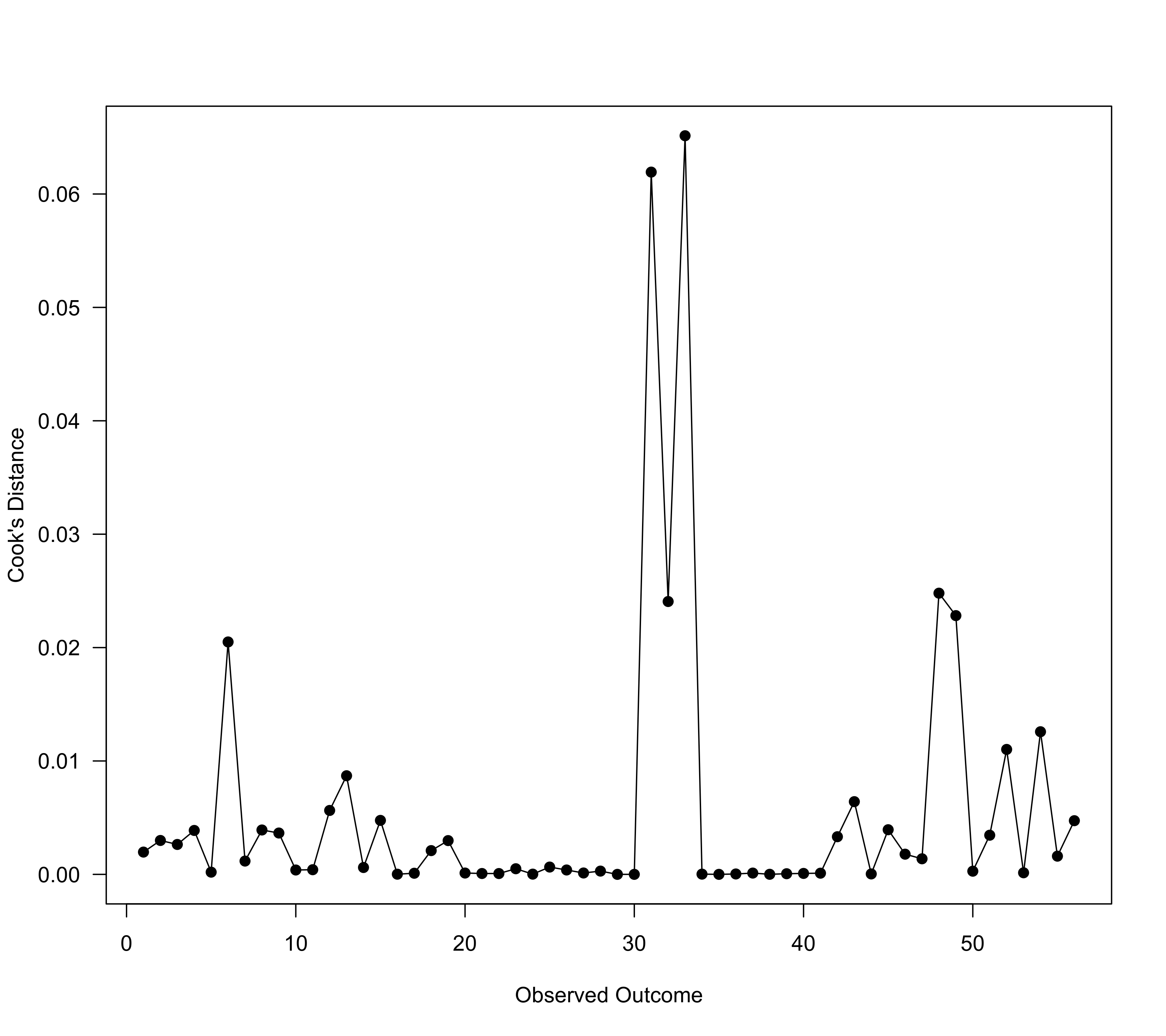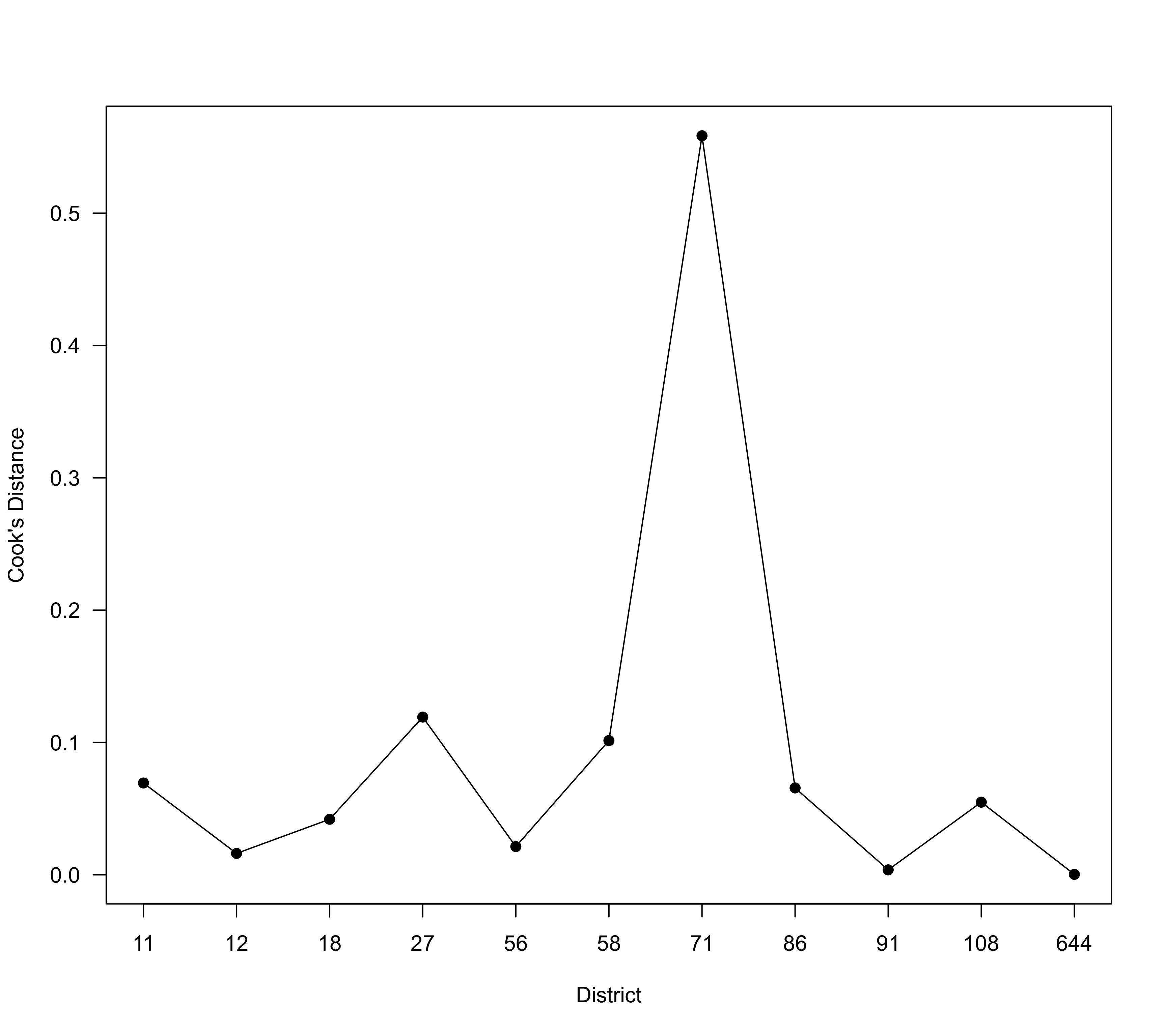Functions to compute various outlier and influential study diagnostics (some of which indicate the influence of deleting one study at a time on the model fit or the fitted/residual values) for objects of class "rma.mv".

# S3 method for rma.mv
cooks.distance(model, progbar=FALSE, cluster,
reestimate=TRUE, parallel="no", ncpus=1, cl, ...)

# S3 method for rma.mv
dfbetas(model, progbar=FALSE, cluster,
reestimate=TRUE, parallel="no", ncpus=1, cl, ...)

# S3 method for rma.mv
hatvalues(model, type="diagonal", ...)

## Arguments

model

an object of class "rma.mv".

progbar

logical to specify whether a progress bar should be shown (the default is FALSE).

cluster

optional vector to specify a clustering variable to use for computing the Cook's distances or DFBETAS values. If unspecified, these measures are computed for the individual observed effect sizes or outcomes.

reestimate

logical to specify whether variance/correlation components should be re-estimated after deletion of the $$i\textrm{th}$$ case (the default is TRUE).

parallel

character string to specify whether parallel processing should be used (the default is "no"). For parallel processing, set to either "snow" or "multicore". See ‘Note’.

ncpus

integer to specify the number of processes to use in the parallel processing.

cl

optional cluster to use if parallel="snow". If unspecified, a cluster on the local machine is created for the duration of the call.

type

character string to specify whether only the diagonal of the hat matrix ("diagonal") or the entire hat matrix ("matrix") should be returned.

...

other arguments.

## Details

The term ‘case’ below refers to a particular row from the dataset used in the model fitting (when argument cluster is not specified) or each level of the variable specified via cluster.

Cook's distance for the $$i\textrm{th}$$ case can be interpreted as the Mahalanobis distance between the entire set of predicted values once with the $$i\textrm{th}$$ case included and once with the $$i\textrm{th}$$ case excluded from the model fitting.

The DFBETAS value(s) essentially indicate(s) how many standard deviations the estimated coefficient(s) change(s) after excluding the $$i\textrm{th}$$ case from the model fitting.

## Value

The cooks.distance function returns a vector. The dfbetas function returns a data frame. The hatvalues function returns either a vector with the diagonal elements of the hat matrix or the entire hat matrix.

## Note

The variable specified via cluster is assumed to be of the same length as the data originally passed to the rma.mv function (and if the data argument was used in the original model fit, then the variable will be searched for within this data frame first). Any subsetting and removal of studies with missing values that was applied during the model fitting is also automatically applied to the variable specified via the cluster argument.

Leave-one-out diagnostics are calculated by refitting the model $$k$$ times (where $$k$$ denotes the number of cases). Depending on how large $$k$$ is, it may take a few moments to finish the calculations. For complex models fitted with rma.mv, this can become computationally expensive.

On machines with multiple cores, one can try to speed things up by delegating the model fitting to separate worker processes, that is, by setting parallel="snow" or parallel="multicore" and ncpus to some value larger than 1. Parallel processing makes use of the parallel package, using the makePSOCKcluster and parLapply functions when parallel="snow" or using mclapply when parallel="multicore" (the latter only works on Unix/Linux-alikes). With parallel::detectCores(), one can check on the number of available cores on the local machine.

Alternatively (or in addition to using parallel processing), one can also set reestimate=FALSE, in which case any variance/correlation components in the model are not re-estimated after deleting the $$i\textrm{th}$$ case from the dataset. Doing so only yields an approximation to the Cook's distances and DFBETAS values that ignores the influence of the $$i\textrm{th}$$ case on the variance/correlation components, but is considerably faster (and often yields similar results).

It may not be possible to fit the model after deletion of the $$i\textrm{th}$$ case from the dataset. This will result in NA values for that case.

## Author

Wolfgang Viechtbauer wvb@metafor-project.org https://www.metafor-project.org

rstudent for externally standardized residuals and weights for model fitting weights.

## Examples

### copy data from Konstantopoulos (2011) into 'dat'
dat <- dat.konstantopoulos2011

### multilevel random-effects model
res <- rma.mv(yi, vi, random = ~ 1 | district/school, data=dat)
print(res, digits=3)
#>
#> Multivariate Meta-Analysis Model (k = 56; method: REML)
#>
#> Variance Components:
#>
#>            estim   sqrt  nlvls  fixed           factor
#> sigma^2.1  0.065  0.255     11     no         district
#> sigma^2.2  0.033  0.181     56     no  district/school
#>
#> Test for Heterogeneity:
#> Q(df = 55) = 578.864, p-val < .001
#>
#> Model Results:
#>
#> estimate     se   zval   pval  ci.lb  ci.ub
#>    0.185  0.085  2.185  0.029  0.019  0.350  *
#>
#> ---
#> Signif. codes:  0 ‘***’ 0.001 ‘**’ 0.01 ‘*’ 0.05 ‘.’ 0.1 ‘ ’ 1
#>

### Cook's distance for each observed outcome
x <- cooks.distance(res)
x
#>            1            2            3            4            5            6            7
#> 1.965212e-03 2.989768e-03 2.638140e-03 3.873368e-03 1.954562e-04 2.049672e-02 1.172821e-03
#>            8            9           10           11           12           13           14
#> 3.913806e-03 3.646294e-03 3.850068e-04 4.111673e-04 5.636933e-03 8.697463e-03 6.052503e-04
#>           15           16           17           18           19           20           21
#> 4.759729e-03 1.527261e-05 9.905788e-05 2.095987e-03 2.978160e-03 1.173555e-04 7.600104e-05
#>           22           23           24           25           26           27           28
#> 7.315289e-05 4.966021e-04 2.024080e-05 6.448093e-04 3.866063e-04 1.220054e-04 2.886483e-04
#>           29           30           31           32           33           34           35
#> 7.676490e-08 1.391702e-06 6.193156e-02 2.406239e-02 6.513879e-02 2.075376e-05 8.763819e-07
#>           36           37           38           39           40           41           42
#> 3.485720e-05 1.141739e-04 6.503505e-06 5.671804e-05 8.326350e-05 1.048009e-04 3.320742e-03
#>           43           44           45           46           47           48           49
#> 6.412251e-03 3.831098e-05 3.937144e-03 1.780433e-03 1.374408e-03 2.479767e-02 2.281846e-02
#>           50           51           52           53           54           55           56
#> 2.803636e-04 3.453698e-03 1.101867e-02 1.405349e-04 1.257869e-02 1.606173e-03 4.733739e-03
plot(x, type="o", pch=19, xlab="Observed Outcome", ylab="Cook's Distance")### Cook's distance for each district
x <- cooks.distance(res, cluster=district)
x
#>           11           12           18           27           56           58           71
#> 0.0693784140 0.0161880606 0.0419849759 0.1192089452 0.0213566370 0.1014506985 0.5585174853
#>           86           91          108          644
#> 0.0656500671 0.0037438517 0.0548839759 0.0003441647
plot(x, type="o", pch=19, xlab="District", ylab="Cook's Distance", xaxt="n")
axis(side=1, at=seq_along(x), labels=as.numeric(names(x)))### hat values
hatvalues(res)
#>           1           2           3           4           5           6           7           8
#> 0.018246414 0.018246414 0.015562154 0.015562154 0.024305990 0.024305990 0.023796820 0.020021978
#>           9          10          11          12          13          14          15          16
#> 0.028628711 0.021068630 0.035668057 0.018288352 0.026253550 0.026253550 0.024271478 0.018700077
#>          17          18          19          20          21          22          23          24
#> 0.024347299 0.025637683 0.026335563 0.008149594 0.008470846 0.008307115 0.007851819 0.008149594
#>          25          26          27          28          29          30          31          32
#> 0.007997936 0.011094988 0.010815774 0.010815774 0.010815774 0.010815774 0.027875751 0.030425177
#>          33          34          35          36          37          38          39          40
#> 0.031137102 0.012900198 0.012900198 0.012900198 0.012900198 0.012900198 0.012900198 0.012900198
#>          41          42          43          44          45          46          47          48
#> 0.012900198 0.016836925 0.016451963 0.016836925 0.017240335 0.015732539 0.015732539 0.018231717
#>          49          50          51          52          53          54          55          56
#> 0.018231717 0.018522325 0.018522325 0.018522325 0.017484652 0.018246600 0.020990821 0.020990821